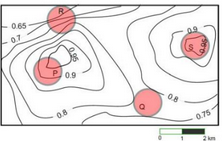# GATE | GATE-CS-2017 (Set 2) | Question 10

An air pressure contour line join the locations in the region having the same atmospheric pressure. The following is the air pressure contour of a geographical region. The contour line are shown as 0.05 bar intervals in this plot.If the probability of thunderstorm is given by how fast air pressure rises or drops over a region. Which of the following region is most likely to have thunderstorm.
(A) P
(B) Q
(C) R
(D) S

Explanation: Region R has maximum air pressure variation as it  which has maximum number of lines crossing the area. Therefore option C is correct

Alternate Solution

We have to see for the region which has maximum change in air pressure for thunderstorm.
In region P – the air pressure changes only once by 0.05 as only 2 lines are crossing the region. The first line with the air pressure 0.95 and the next one is with pressure 0.9.
In region Q – No line crosses the region which implies that the air pressure doesn’t change.
In region R – the air pressure changes at 4 places resulting in maximum change in air pressure in the whole region. The minimum pressure in R is 0.65 and the maximum is 0.8.
In region S – the air pressure in the region in 0.95 and other contouring line is outside the region thus no change in air pressure within the region.
Thus the probability of thunderstorm is maximum in region R.

This solution is contributed by Parul Sharma.

Quiz of this Question

My Personal Notes arrow_drop_up

Article Tags :

Be the First to upvote.

Please write to us at contribute@geeksforgeeks.org to report any issue with the above content.